# If, And, Or functions in Excel

##### How to work with If, And and Or functions in MS Excel?

In the previous post, we learnt about Sumproduct, SumsQ function in Excel. In this post, we shall learn about Logical functions such as If, And and Or in Excel.

### If Function

IF is a conditional function that returns one value based on whether the condition is valid or not. In below example, F column should have a value (Adult or Minor) based on E column data (Age), so the formula we have written in F column is '=IF(E3>18, "Adult", "Minor")'.

ie

For row 3, it checks if E3 column value is more than 18 then writes 'Adult' else 'Minor'. The same formula has been copied for other rows. The result is below.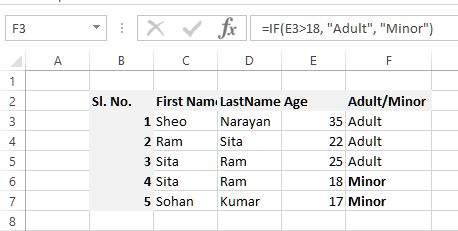### Nested IF Function

IF function can be nested within another IF. Look at below example.

In I3 cell, the 1st parameter is the IF function is again an IF function that returns TRUE/FALSE based on E3 value and then writes 'Major' or 'Minor' in the cell.

When we copy the same formula to other rows, the result would be like this.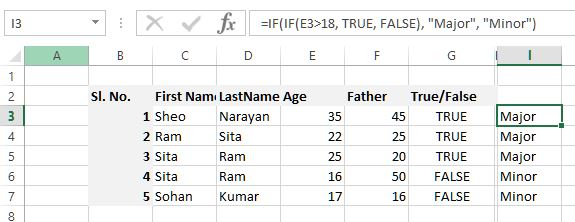### AND Function

AND function returns

1. TRUE if all conditions are valid
2. FALSE even if one condition is invalid
Look at the example below. Here we have written '=AND(E3>18,F3>18)' in F3 cell that checks the value of E3 and F3. If both are greater than 18 then writes TRUE else FALSE.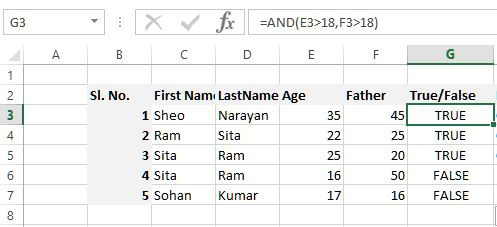The return value of 'AND' function can be used inside another function as well. Look at the example below.
In G3 cell we have written '=IF(AND(E3>18,F3>18), "Correct", "Incorrect")', it

• first checks E3>18 and F3>18, if both are valid then returns TRUE otherwise FALSE
• IF function then checks the return value of AND function and if it is TRUE, writes 'Major' otherwise 'Minor'.
The same formula has been copied into G column cells as well and here is the result.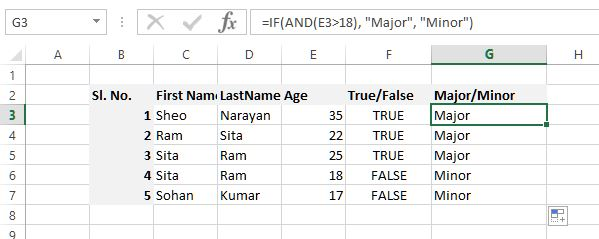### OR Function

OR function returns

• TRUE if any of the conidtion is valid
• FALSE if all conditions are invalid
In below example, G3 cell contains '=OR(E3>18,F3>18)' formula. This checks the value of E3 and F3 and if both are greater than 18, it returns TRUE otherwise FALSE.
The result is below.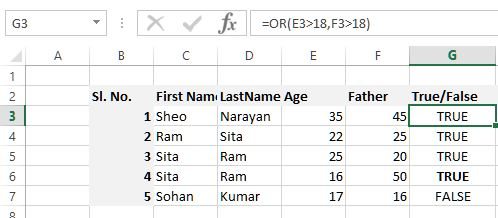The return value of OR can also be used within another function. Look at below example.
Cell H3 contains '=IF(OR(E3>18,F3>18), "Correct", "Incorrect")' formula. Here the return value of OR function is used in IF condition and then 'Correct' or 'Incorrect' value is returned.
The result is below.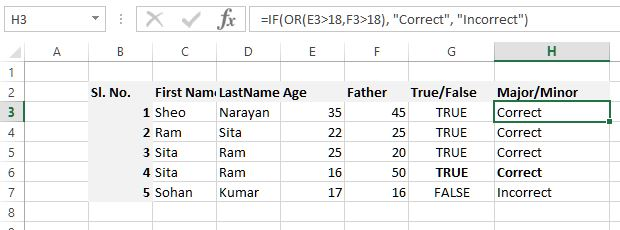Look at H6 cell, here E6 returns TRUE but F6 returns FALSE. Even if 1 condition is FALSE, OR function returns TRUE and the result is 'Correct'.